Successfully reported this slideshow.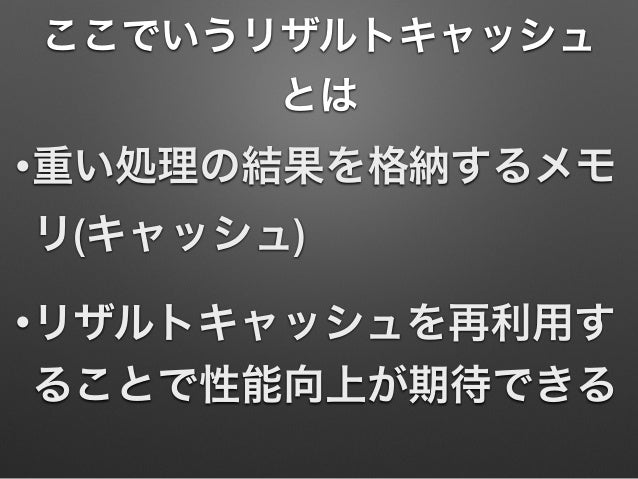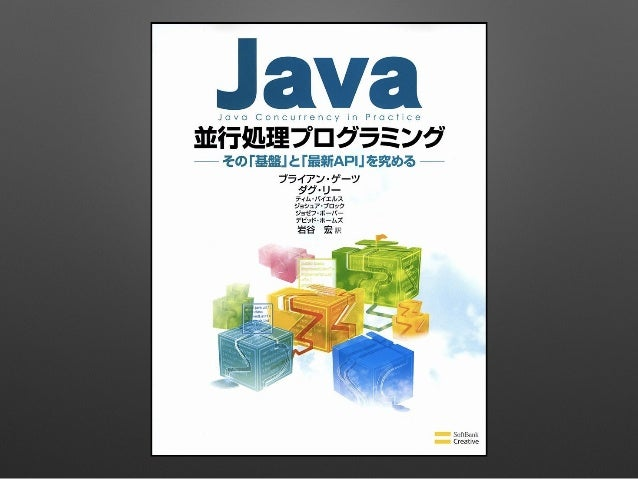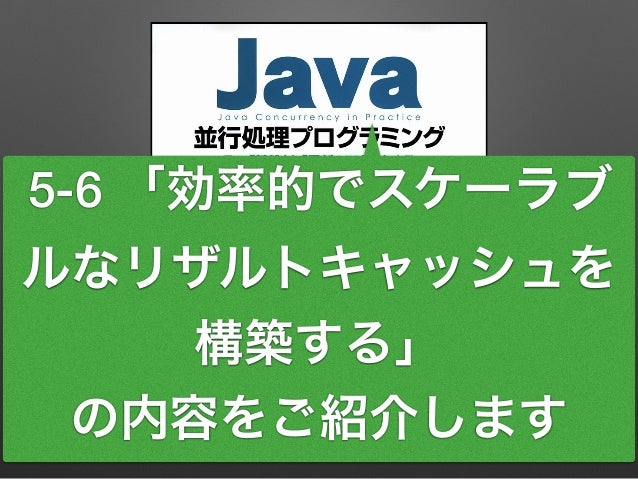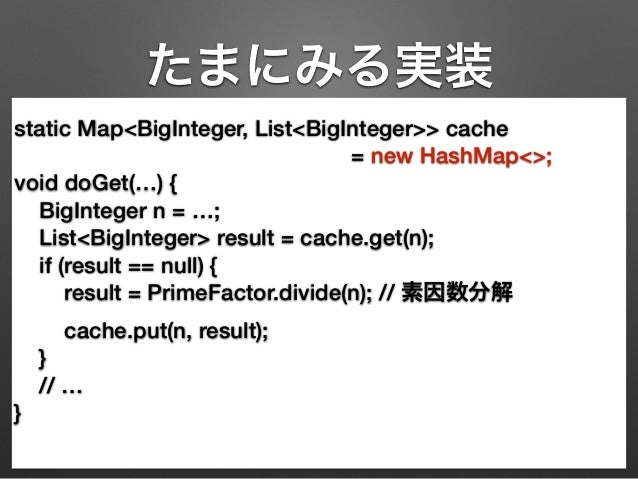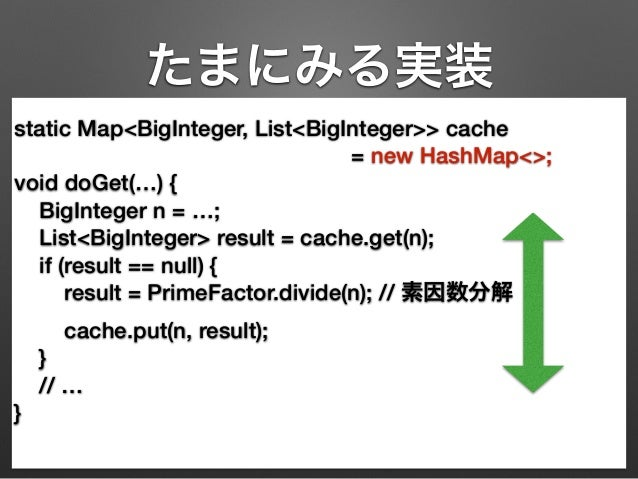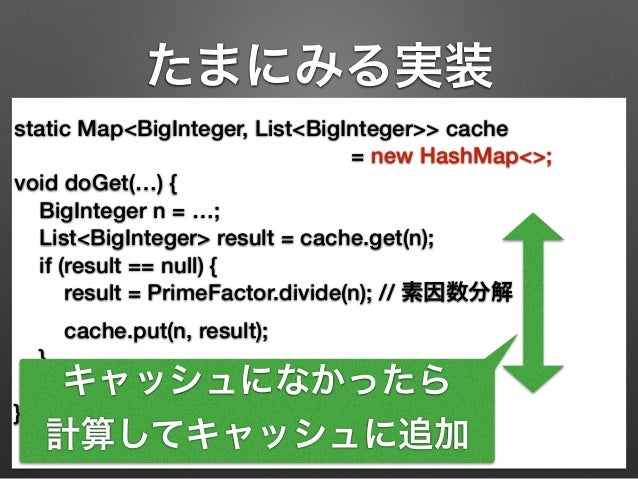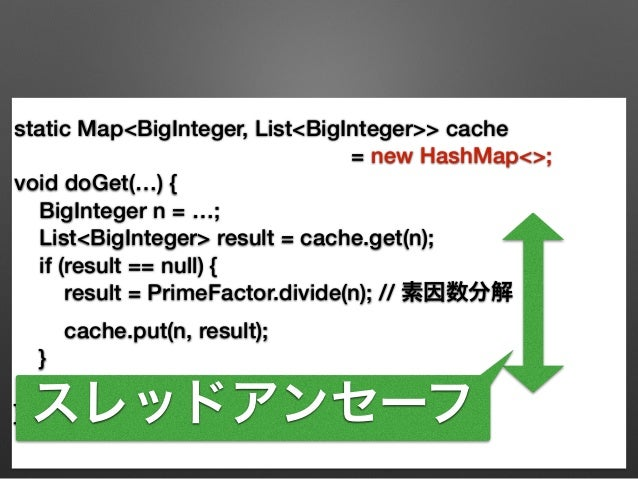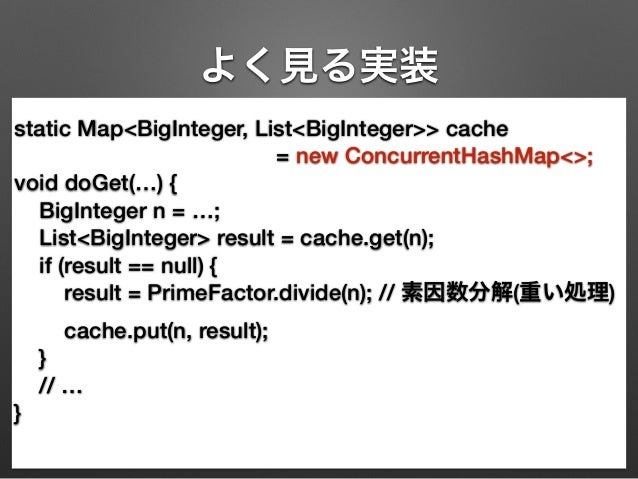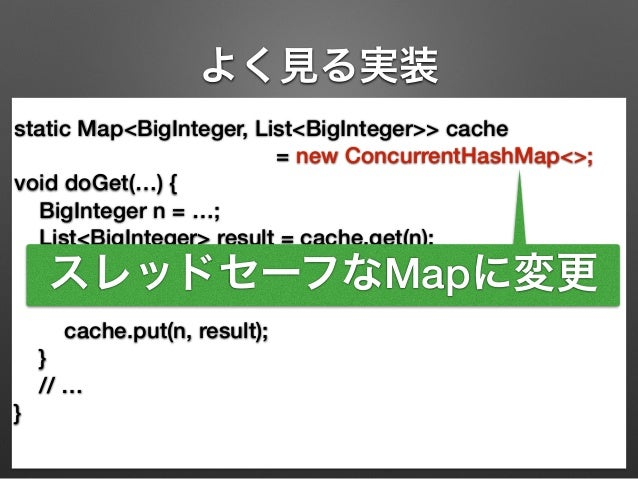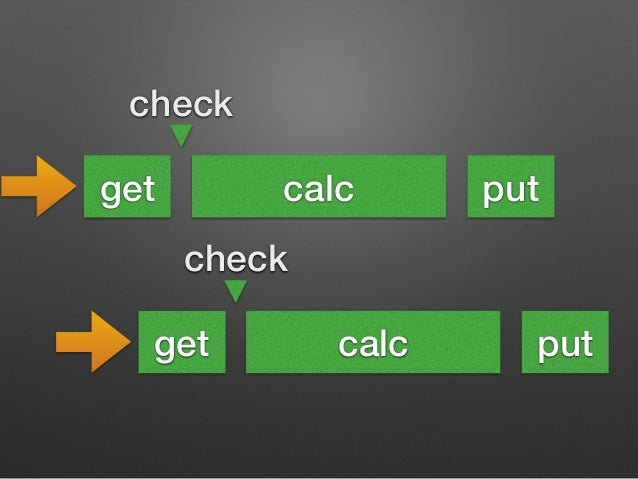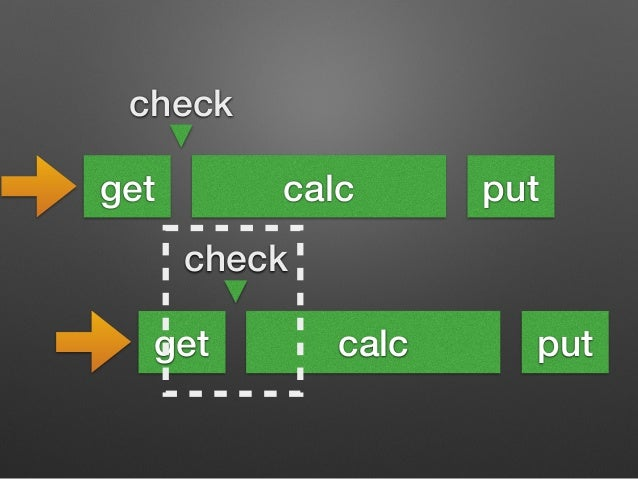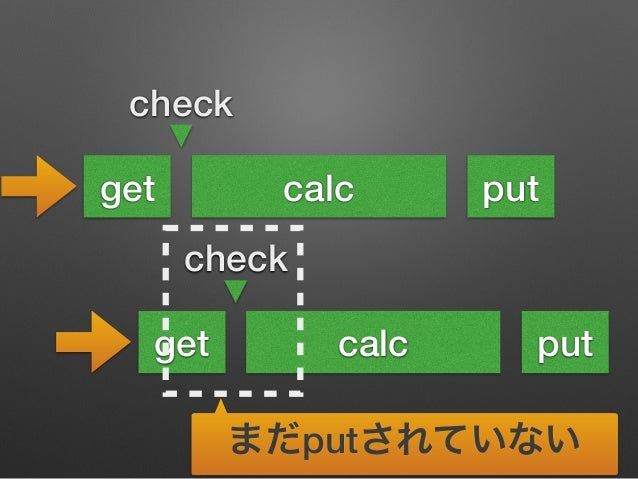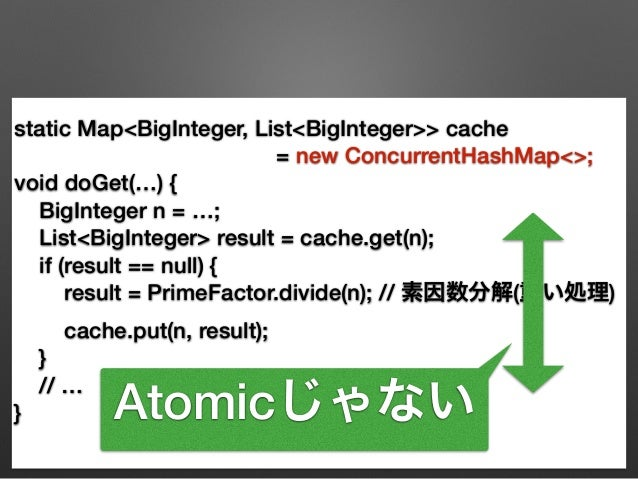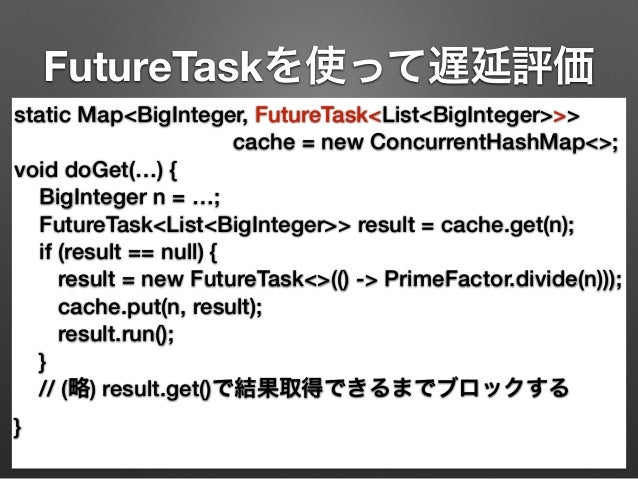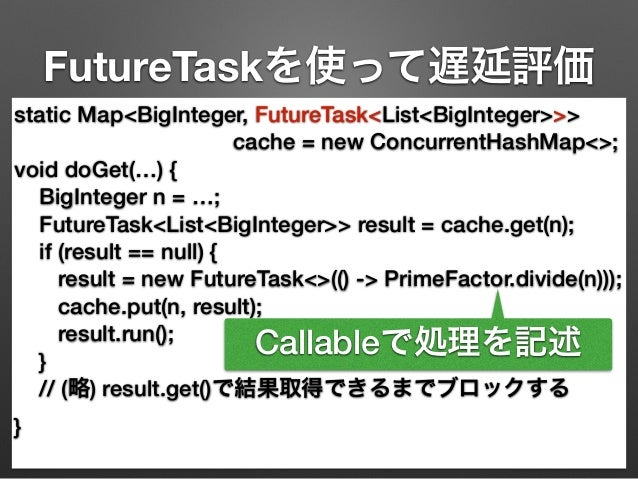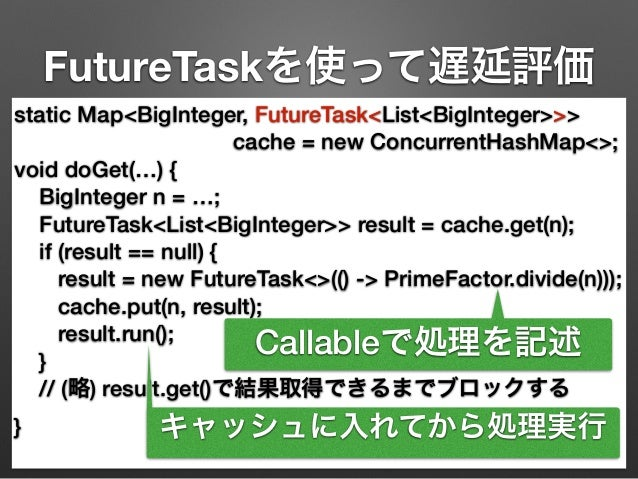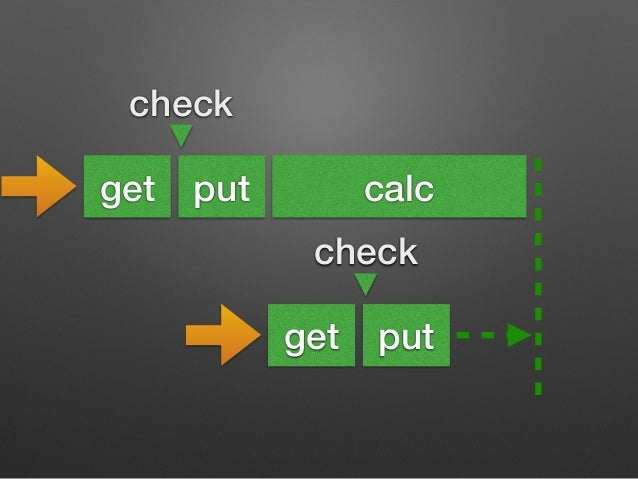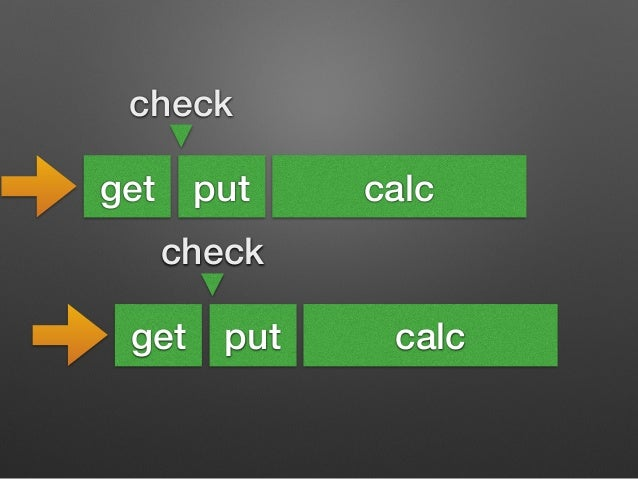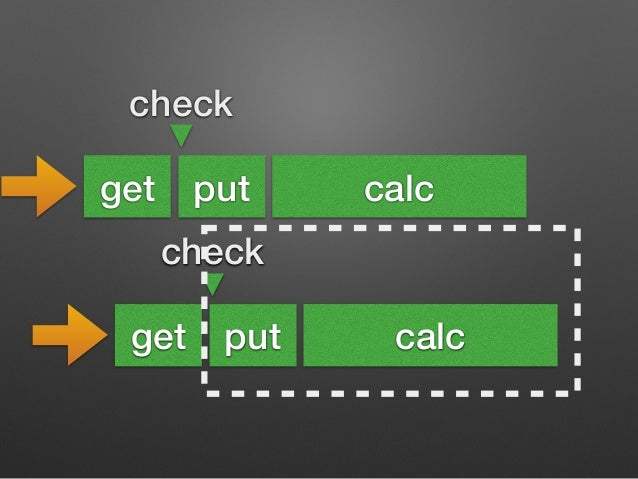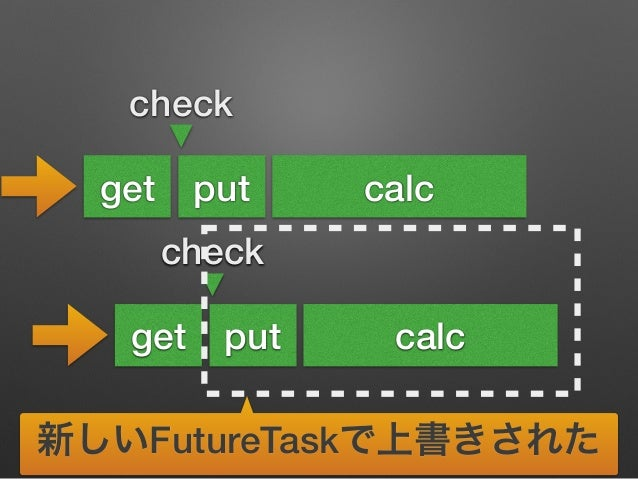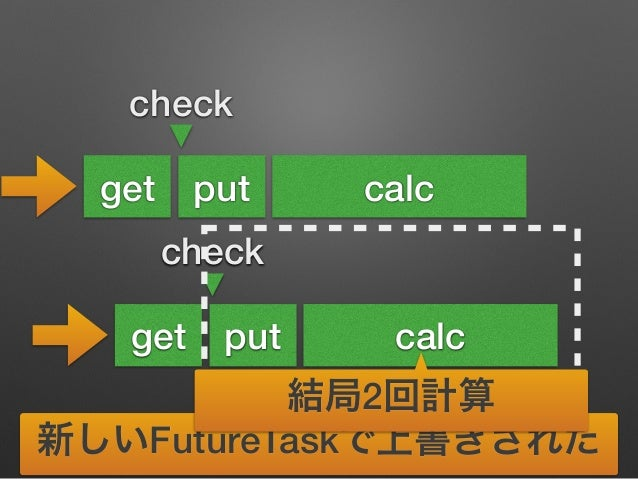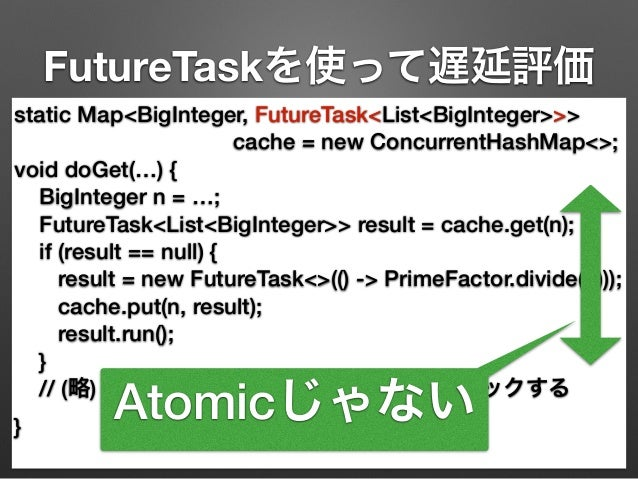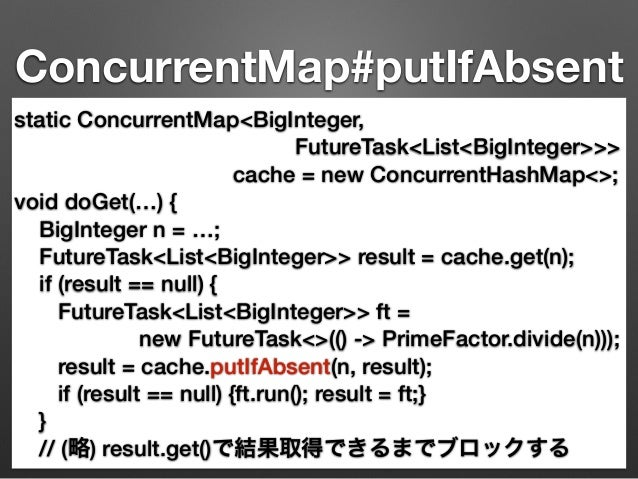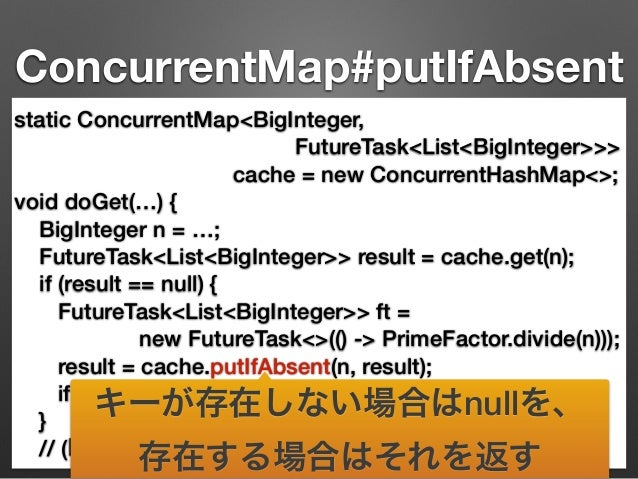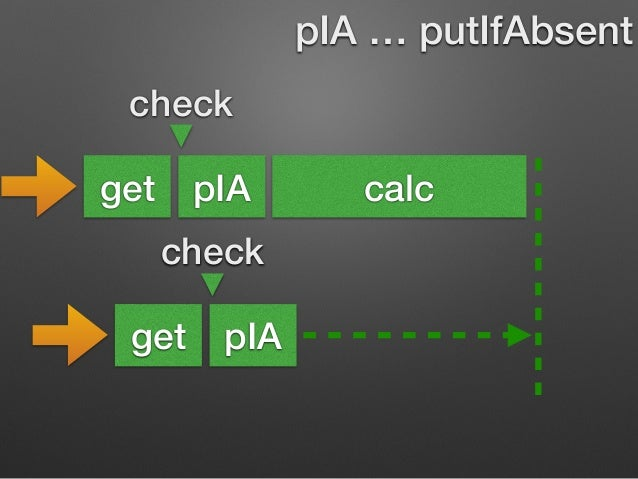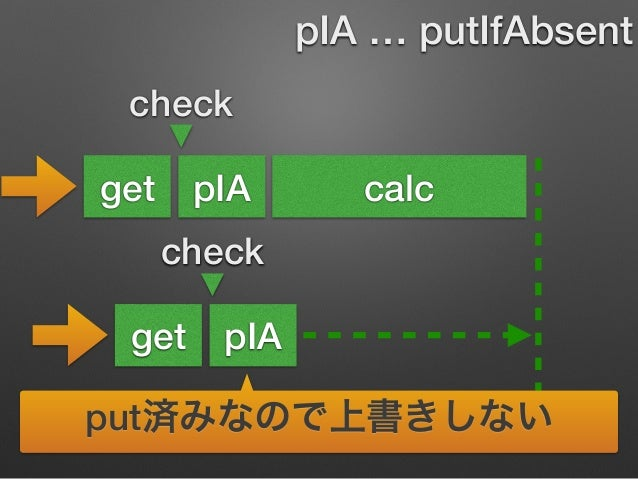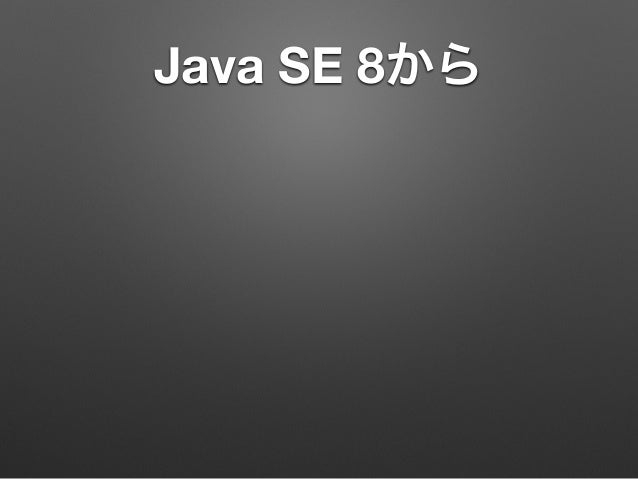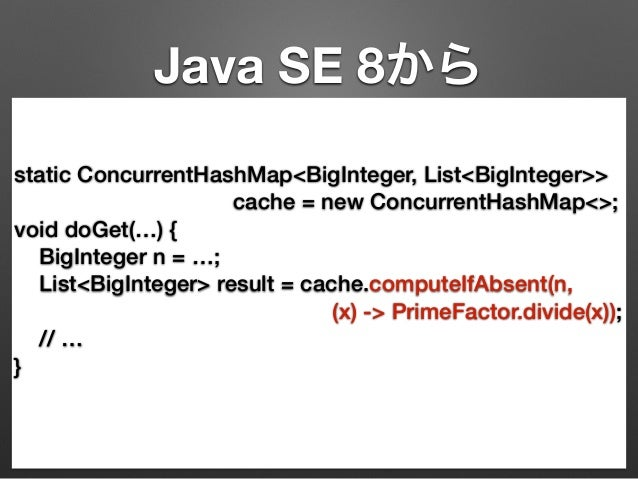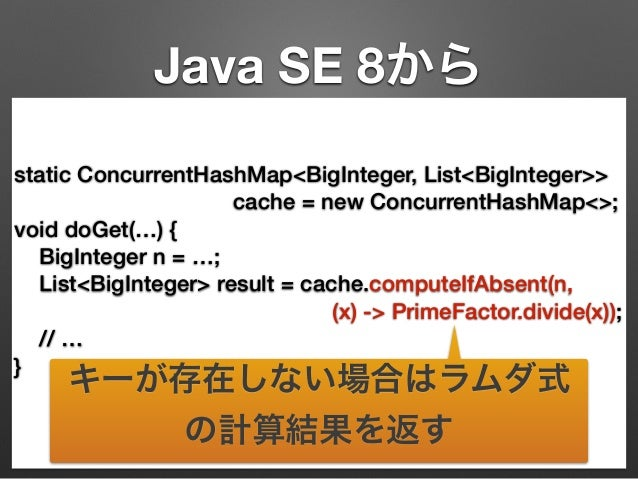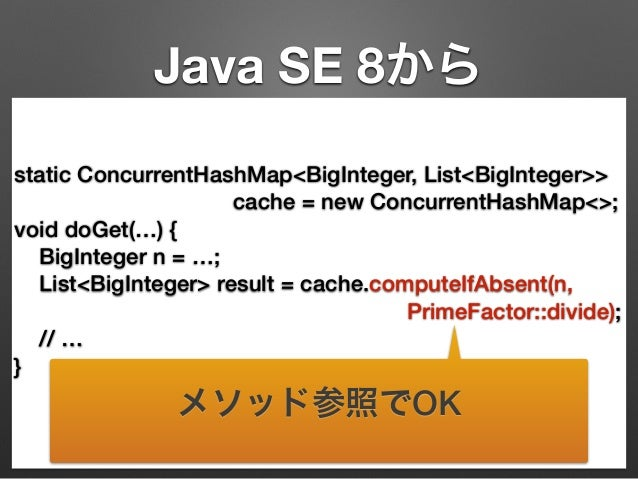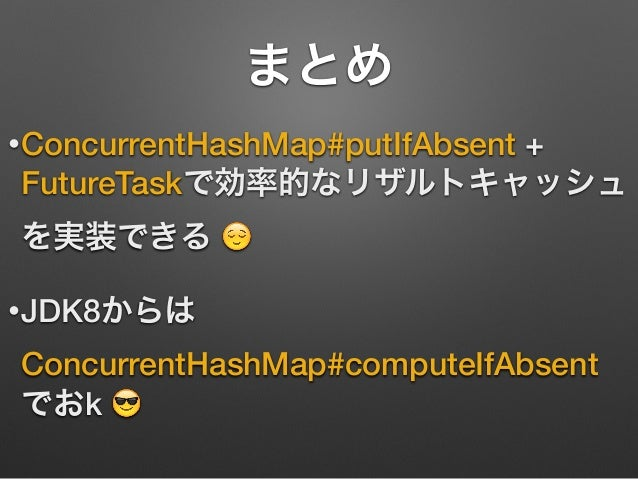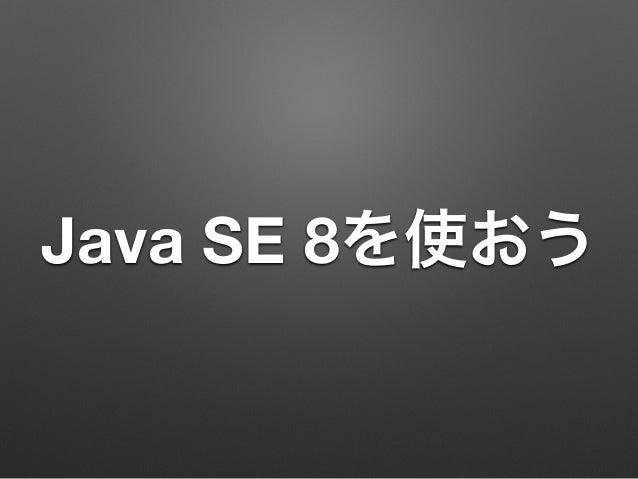Upcoming SlideShare
×
• Full Name
Comment goes here.

Are you sure you want to Yes No• Be the first to comment

### そんなリザルトキャッシュで大丈夫か? #jjug

1. 1. そんなリザルトキャッシュ で大丈夫か？ @making 槙 俊明 2014-09-17
2. 2. ここでいうリザルトキャッシュ とは •重い処理の結果を格納するメモ リ(キャッシュ) •リザルトキャッシュを再利用す ることで性能向上が期待できる
3. 3. あなたのリザルトキャッシュ •スレッドセーフですか？ •スケーラブルですか？
4. 4. 5-6 「効率的でスケーラブ ルなリザルトキャッシュを 構築する」 の内容をご紹介します
5. 5. たまにみる実装
6. 6. たまにみる実装 static Map<BigInteger, List<BigInteger>> cache = new HashMap<>; void doGet(…) { BigInteger n = …; List<BigInteger> result = cache.get(n); if (result == null) { result = PrimeFactor.divide(n); // 素因数分解 cache.put(n, result); } // … }
7. 7. たまにみる実装 static Map<BigInteger, List<BigInteger>> cache = new HashMap<>; void doGet(…) { BigInteger n = …; List<BigInteger> result = cache.get(n); if (result == null) { result = PrimeFactor.divide(n); // 素因数分解 cache.put(n, result); } // … }
8. 8. たまにみる実装 static Map<BigInteger, List<BigInteger>> cache = new HashMap<>; void doGet(…) { BigInteger n = …; List<BigInteger> result = cache.get(n); if (result == null) { result = PrimeFactor.divide(n); // 素因数分解 cache.put(n, result); } // … } キャッシュになかったら 計算してキャッシュに追加
9. 9.
10. 10. static Map<BigInteger, List<BigInteger>> cache = new HashMap<>; void doGet(…) { BigInteger n = …; List<BigInteger> result = cache.get(n); if (result == null) { result = PrimeFactor.divide(n); // 素因数分解 cache.put(n, result); } // … } スレッドアンセーフ
11. 11. よく見る実装 static Map<BigInteger, List<BigInteger>> cache = new ConcurrentHashMap<>; void doGet(…) { BigInteger n = …; List<BigInteger> result = cache.get(n); if (result == null) { result = PrimeFactor.divide(n); // 素因数分解(重い処理) cache.put(n, result); } // … }
12. 12. よく見る実装 static Map<BigInteger, List<BigInteger>> cache = new ConcurrentHashMap<>; void doGet(…) { BigInteger n = …; List<BigInteger> result = cache.get(n); if (result == null) { スレッドセーフなMapに変更 result = PrimeFactor.divide(n); // 素因数分解(重い処理) cache.put(n, result); } // … }
13. 13. Demo
14. 14.
15. 15. check get calc put check get calc put
16. 16. check get calc put check get calc put
17. 17. check get calc put check get calc put まだputされていない
18. 18. static Map<BigInteger, List<BigInteger>> cache = new ConcurrentHashMap<>; void doGet(…) { BigInteger n = …; List<BigInteger> result = cache.get(n); if (result == null) { result = PrimeFactor.divide(n); // 素因数分解(重い処理) cache.put(n, result); } // … } Atomicじゃない
20. 20. FutureTaskを使って遅延評価 static Map<BigInteger, FutureTask<List<BigInteger>>> cache = new ConcurrentHashMap<>; void doGet(…) { BigInteger n = …; FutureTask<List<BigInteger>> result = cache.get(n); if (result == null) { result = new FutureTask<>(() -> PrimeFactor.divide(n))); cache.put(n, result); result.run(); } // (略) result.get()で結果取得できるまでブロックする }
21. 21. FutureTaskを使って遅延評価 static Map<BigInteger, FutureTask<List<BigInteger>>> cache = new ConcurrentHashMap<>; void doGet(…) { BigInteger n = …; FutureTask<List<BigInteger>> result = cache.get(n); if (result == null) { result = new FutureTask<>(() -> PrimeFactor.divide(n))); cache.put(n, result); result.run(); Callableで処理を記述 } // (略) result.get()で結果取得できるまでブロックする }
22. 22. FutureTaskを使って遅延評価 static Map<BigInteger, FutureTask<List<BigInteger>>> cache = new ConcurrentHashMap<>; void doGet(…) { BigInteger n = …; FutureTask<List<BigInteger>> result = cache.get(n); if (result == null) { result = new FutureTask<>(() -> PrimeFactor.divide(n))); cache.put(n, result); result.run(); Callableで処理を記述 } // (略) result.get()で結果取得できるまでブロックする } キャッシュに入れてから処理実行
23. 23. check get put calc check get put
24. 24. Demo
25. 25.
26. 26. check get put calc check get put calc
27. 27. check get put calc check get put calc
28. 28. check get put calc check get put calc 新しいFutureTaskで上書きされた
29. 29. check get put calc check get put calc 結局2回計算 新しいFutureTaskで上書きされた
30. 30. FutureTaskを使って遅延評価 static Map<BigInteger, FutureTask<List<BigInteger>>> cache = new ConcurrentHashMap<>; void doGet(…) { BigInteger n = …; FutureTask<List<BigInteger>> result = cache.get(n); if (result == null) { result = new FutureTask<>(() -> PrimeFactor.divide(n))); cache.put(n, result); result.run(); } // (略) result.get()で結果取得できるまでブロックする } Atomicじゃない
31. 31. ConcurrentMap#putIfAbsent
32. 32. ConcurrentMap#putIfAbsent static ConcurrentMap<BigInteger, FutureTask<List<BigInteger>>> cache = new ConcurrentHashMap<>; void doGet(…) { BigInteger n = …; FutureTask<List<BigInteger>> result = cache.get(n); if (result == null) { FutureTask<List<BigInteger>> ft = new FutureTask<>(() -> PrimeFactor.divide(n))); result = cache.putIfAbsent(n, result); if (result == null) {ft.run(); result = ft;} } // (略) result.get()で結果取得できるまでブロックする
33. 33. ConcurrentMap#putIfAbsent static ConcurrentMap<BigInteger, FutureTask<List<BigInteger>>> cache = new ConcurrentHashMap<>; void doGet(…) { BigInteger n = …; FutureTask<List<BigInteger>> result = cache.get(n); if (result == null) { FutureTask<List<BigInteger>> ft = new FutureTask<>(() -> PrimeFactor.divide(n))); result = cache.putIfAbsent(n, result); if (result == null) {ft.run(); result = ft;} } // (略) result.get()で結果取得できるまでブロックする キーが存在しない場合はnullを、 存在する場合はそれを返す
34. 34. check get pIA calc check get pIA pIA … putIfAbsent
35. 35. check get pIA calc check get pIA pIA … putIfAbsent put済みなので上書きしない
36. 36. Demo
37. 37.
38. 38. でも面倒くさいね！ 
39. 39. Java SE 8から
40. 40. Java SE 8から static ConcurrentHashMap<BigInteger, List<BigInteger>> cache = new ConcurrentHashMap<>; void doGet(…) { BigInteger n = …; List<BigInteger> result = cache.computeIfAbsent(n, (x) -> PrimeFactor.divide(x)); // … }
41. 41. Java SE 8から static ConcurrentHashMap<BigInteger, List<BigInteger>> cache = new ConcurrentHashMap<>; void doGet(…) { BigInteger n = …; List<BigInteger> result = cache.computeIfAbsent(n, (x) -> PrimeFactor.divide(x)); // … } キーが存在しない場合はラムダ式 の計算結果を返す
42. 42. Java SE 8から static ConcurrentHashMap<BigInteger, List<BigInteger>> cache = new ConcurrentHashMap<>; void doGet(…) { BigInteger n = …; List<BigInteger> result = cache.computeIfAbsent(n, PrimeFactor::divide); // … } メソッド参照でOK
43. 43. Demo
44. 44. Cool! 
45. 45. まとめ •ConcurrentHashMap#putIfAbsent + FutureTaskで効率的なリザルトキャッシュ を実装できる  •JDK8からは ConcurrentHashMap#computeIfAbsent でおk 
46. 46. Java SE 8を使おう
47. 47. ご清聴 ありがとうございました

### Be the first to comment

•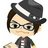#### takecy

Sep. 17, 2014
•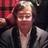#### KanSakamoto

Sep. 17, 2014
•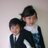#### KeisukeTkeuchi

Sep. 17, 2014
•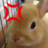#### akisei

Sep. 17, 2014
•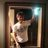#### hashimotoms

Sep. 17, 2014
•#### fukuihi

Sep. 17, 2014
•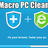#### AijazAhmed10

Sep. 18, 2014
•#### ohtsuchi

Sep. 18, 2014
•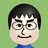#### takehiroinoue127

Sep. 18, 2014
•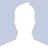#### YoshioHara

Sep. 18, 2014
•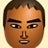#### matsumana0101

Sep. 18, 2014
•#### hiro_ist

Sep. 19, 2014
•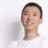#### mitsuruogawa33

Sep. 20, 2014
•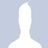#### TakashiYasukawa1

Sep. 23, 2014
•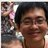#### itokami1123

Sep. 28, 2014
•#### masakazuyamanaka

Sep. 29, 2014
•#### orekyuu

Oct. 5, 2014
•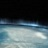#### TsukasaTamaru

Nov. 22, 2014
•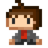#### sfus

Sep. 17, 2015
•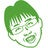Oct. 7, 2015

Total views

10,344

On Slideshare

0

From embeds

0

Number of embeds

2,198

26

Shares

0